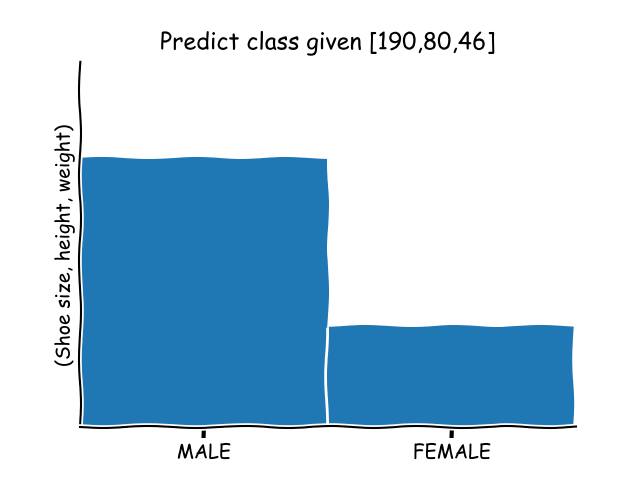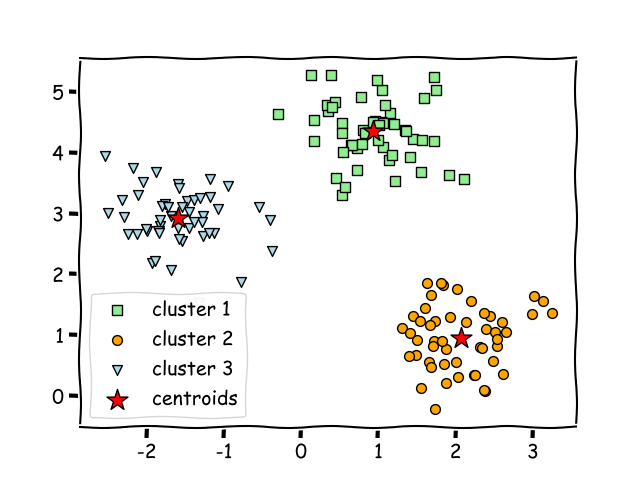Data science is considered to be one of the most exciting fields in which you could work due to the fact that it combines both advanced statistical and quantitative skills with real-world programming ability.

There are available a multitude of algorithms that have the ability to assist data scientists in their research and assessments. Even though at first everything might seem complicated, gaining understanding about the two most commonly used algorithms will make your approach to machine learning smoother.

Related course:

## K-means clustering vs k-nearest neighbors

The two most commonly used algorithms in machine learning are K-means clustering and k-nearest neighbors algorithm.

Often those two are confused with each other due to the presence of the `k` letter, but in reality, those algorithms are slightly different from each other.

Thus, K-means clustering represents an unsupervised algorithm, mainly used for clustering, while KNN is a supervised learning algorithm used for classification.

### K-nearest neighbors

Being a supervised classification algorithm, K-nearest neighbors needs labelled data to train on.

With the given data, KNN can classify new, unlabelled data by analysis of the `k` number of the nearest data points. Thus, the variable `k` is considered to be a parameter that will be established by the machine learning engineer.

Thus, k-kmeans needs training data to make predictions.### K-means

On the other hand, K-means clustering represents an unsupervised clustering algorithm that needs unlabelled data to train.K-means clustering is able to gradually learn how to cluster the unlabelled points into groups by analysis of the mean distance of said points. In this case, the variable `k` depicts the number of clusters or different groups in which the data will be gathered. The algorithm functions by moving the data in such manner that error function is minimized.

### Differences

K-nearest neighbor algorithm is mainly used for classification and regression of given data when the attribute is already known.

This stands as a major difference between the two algorithms due to the fact that the K-means clustering algorithm is popularly used for scenarios such as getting deeper understanding of demographics, social media trends, marketing strategies evolution and so on.

Hence, KNN and k-means clustering are important algorithms when it comes to machine learning.

But each algorithm Is meant to deal with different problems and provide different meaning of what the variable `k` stands for.

• KNN represents a supervised classification algorithm that will give new data points accordingly to the `k` number or the closest data points,
• while k-means clustering is an unsupervised clustering algorithm that gathers and groups data into `k` number of clusters.

Anyhow, there is a common aspect which can be encountered in both algorithms: KNN and k-means clustering represent distance-based algorithms that rely on a metric.